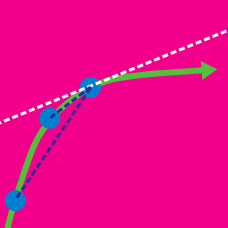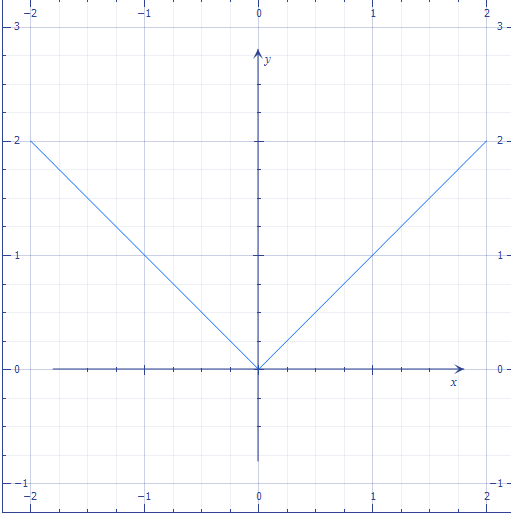Calculus

# Differentiability: Level 2 ChallengesAbove shows the graph of $f(x) = | x|$, what is the value of $f'(0)$?

A motorist is driving down the freeway that has a speed limit of $60$ MPH. The police car he passes at $10\text{:}30$ AM records him as going $60$ MPH, so he isn't pulled over. Two hours and $156$ miles later, he passes another police car, again registering as traveling at $60$ MPH, so once again he is not pulled over. At some point during the trip, was he speeding? If so, you know this due to which theorem?To use a coffee machine, you fill a drinking cup with water, pour the water into the reservoir, and then put your cup under the spout. Once the water is heated, it flows through coffee grounds and into your cup. Once you use a cup to drink, it is coated with sugar, coffee, and other residues that would ruin the internal workings of the coffee machine, so you cannot reuse the cup.

If instead, you use two cups, one that you always use to fill the coffee machine with water, and a second that you always use to collect the coffee, you can limit the number of coffee cups you have to use in total to two.

Question: Which of the following functions describes your fractional savings in the total number of coffee cups you've had to use as a function of $c$, the number of cups of coffee you've made?

Given that the equation of the tangent to the curve $(y-2)^2=x,$ which is parallel to the line $x-2y=4,$ can be expressed in the form $Ax-By+C=0,$ where $A, B$ and $C$ are coprime, positive integers, find the value of $A-B+C.$

True or false?:

"There exists a number that is exactly one more than its cube."

×